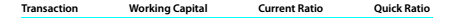Chapter 9, Problem 9.3.2P

Chapter
Section
Textbook Problem

Effect of transactions on current position analysis Data pertaining to the current position of Newlan Company are as follows:InstructionsList the following captions on a sheet of paper:.Compute the working capital, the current ratio, and the quick ratio after each of the following transactions, and record the results in the appropriate columns. Consider each transaction separately and assume that only that transaction affects the data given above. Round to one decimal place. a.Sold temporary investments for cash at no gain or loss, $50,000. b. Paid accounts payable,$40,000. c.Purchased goods on account, $75,000. d. Paid notes payable,$30,000. e. Declared a cash dividend, $15,000. f.Declared a stock dividend on common stock,$24,000. g. Borrowed cash from bank on a long-term note, $150,000. h. Received cash on account,$72,000. i.Issued additional shares of stock for cash, $300,000. j.Paid cash for prepaid expenses.$10,000.

To determine

Introduction:

Financial Ratios:

Financial ratios are the metrics used to evaluate the liquidity efficiency and profitability and overall performance of the business.

To compute:

Working capital, Current ratio and Quick ratio.

Working capital is the portion of capital used to run day to day activities of the business.It is the difference between current assets and current liabilities. Itmeasures a company's liquidity, operational efficiency and short-term financial health.

The current ratio is a liquidity ratio which measures ability of a business to pay short term dues.It can be calculated as follows:

Current ratio=Current assets Current liabilities

The quick ratio measures short-term liquidity position of a business using its liquid assets.It can be calculated as follows:

Quick ratio=Current assetsInventory Current liabilities

To compute:

Effect of transactions on the current assets, current liabilities and current ratio and quick ratios.

Explanation
 Transactions Effect of transaction working capital current ratio quick ratio opening balances current assets 675,000 current liabilities 250,000 425,000 2.7 1.94 Sold temporary investments at no gain or loss, $50,000 No effect as sale of investment decreased one current asset and increased cash balance current assets 675,000 current liabilities 250,000 425,000 2.7 1.94 Paid accounts payable,$40,000 Decrease in current liability and decrease in current assets current assets 635,000 current liabilities 210,000 425,000 3.0 2.1 Purchased goods on account, $75,000 Increase in inventory and increase in accounts payable current assets 750,000 current liabilities 325,000 425,000 2.3 1.7 Paid notes payable,$30,000. Decrease in current liability and decrease in current assets current assets 645,000 current liabilities 220,000 435,000 2,93 2.0 Declared a cash dividend, \$15,000. Increase current liability current assets 675,000 current liabilities 265,000 410,000 2...

Still sussing out bartleby?

Check out a sample textbook solution.

See a sample solution

The Solution to Your Study Problems

Bartleby provides explanations to thousands of textbook problems written by our experts, many with advanced degrees!

Get Started

Assets are items that are owned by the business and are expected to provide future benefits.

College Accounting, Chapters 1-27 (New in Accounting from Heintz and Parry)

How does a sole proprietorship differ from partnership?

Foundations of Business (MindTap Course List)

MVA Henderson Industries has 500 million of common equity on its balance sheet; its stock price is 60 per share...

Fundamentals of Financial Management, Concise Edition (with Thomson ONE - Business School Edition, 1 term (6 months) Printed Access Card) (MindTap Course List)